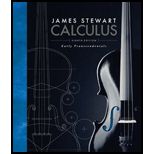# (a) We define the improper integral (over the entire plane ℝ 2 ) I = ∬ R 2 e − ( x 2 + y 2 ) d A = ∫ − ∞ ∞ ∫ − ∞ ∞ e − ( x 2 + y 2 ) d y d x = lim a → ∞ ∬ D a e − ( x 2 + y 2 ) d A where D a is the disk with radius a and center the origin. Show that ∫ − ∞ ∞ ∫ − ∞ ∞ e − ( x 2 + y 2 ) d A = π (b) An equivalent definition of the improper integral in part (a) is ∬ R 2 e − ( x 2 + y 2 ) d A = lim a → ∞ ∬ S a e − ( x 2 + y 2 ) d A where S a is the square with vertices ( ±a, ±a ). Use this to show that ∫ − ∞ ∞ e − x 2 d x ∫ − ∞ ∞ e − y 2 d y = π (c) Deduce that ∫ − ∞ ∞ e − x 2 d x = π (d) By making the change of variable t = 2 x , show that ∫ − ∞ ∞ e − x 2 / 2 d x = 2 π (This is a fundamental result for probability and statistics.)### Calculus: Early Transcendentals

8th Edition
James Stewart
Publisher: Cengage Learning
ISBN: 9781285741550### Calculus: Early Transcendentals

8th Edition
James Stewart
Publisher: Cengage Learning
ISBN: 9781285741550

#### Solutions

Chapter
Section
Chapter 15.3, Problem 40E
Textbook Problem

## Expert Solution

### Want to see the full answer?

Check out a sample textbook solution.See solution

### Want to see this answer and more?

Experts are waiting 24/7 to provide step-by-step solutions in as fast as 30 minutes!*

See Solution

*Response times vary by subject and question complexity. Median response time is 34 minutes and may be longer for new subjects.